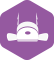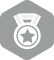### 在matlab中调用VC写的dll的内容失败

VC/MFC > 进程/线程/DLL [问题点数：100分，无满意结帖，结帖人ylmszhanghua]2005年 总版技术专家分年内排行榜第二2009年3月 总版技术专家分月排行榜第二
2009年1月 总版技术专家分月排行榜第二
2005年7月 总版技术专家分月排行榜第二
2005年5月 总版技术专家分月排行榜第二
2005年3月 总版技术专家分月排行榜第二2015年8月优秀小版主
2015年9月优秀小版主
2015年5月优秀小版主
2015年2月论坛优秀版主abzhang2

matlab调用VC++生成的DLL

matlab是一种脚本语言，执行效率肯定没有C语言那样高效，当然matlab支持调用由C语言生成的.dll动态链接库，从而可以达到matlab与C语言混合便宜的效果。  注意：我使用的平台是matlab2016a与vs2015 1、新建一个空...

vs中调用matlab生成的dll 以及一些错误解决办法

VCMatlab混合编程(在VC中调用DLL)

Matlab 作为当今世界上应用最为广泛的数学软件， 具有非常强大的数值计算、数据分析处理、系统分析、图形显示甚至符号运算的功能。已经如生物工程，图像处理，语音处理，雷达探...但是Matlab 强大的功能只能它所...

VS或VC调用matlab程序dll路径配置及错误分析

1：在matlab中选择compiler。  命令行窗口输入：mex -setup   按照提示选择vc6.0.由于使用的是matlab7，不识别vs2010和2008，无奈有装的vc6.0。但听说最新版的matlab可以识别。 2：选择builder.  ...

MATLABVC混编DLL加载问题

（个人总结）VC++调用Matlab生成的dll图文详解

c++调用matlab函数 - dll方式

matlab生成dll

﻿﻿ 实验室的一个项目需要调用matlab程序。经过再三考虑，决定使用vc调用...1：在matlab中选择compiler。  命令行窗口输入：mex -setup   按照提示选择vc6.0.由于使用的是matlab7，不识别vs2010和2008，无

matlab和c++混合编程---方法和步骤

Matlab调用C/C++代码及C/C++调用m文件两方面,深入地研究了它们之间混合编程的原理和实现机制,并且给出了具体条件下的混合编程方法和步骤。实验表明,给出的Matlab与C/C++混合编程接口及应用方法是有效、实用的。

1. 首先要有被调试DLL的源代码工程（假设名字为DLL工程）； 2. 其次要有调用这个DLL的EXE，有没有该EXE的工程没关系，但一定要有该EXE，这里假设该exe为EXE_DLL.exe...4. 在DLL工程的源代码设置好断点，直接F5即可。

c++调用matlab生成的Dll动态连接库

c++调用matlab生成的Dll动态连接库 实验平台：  matlab 7.0(R2009a) VC++6.0 思路： 1. 设置matlab的编译器，使用外部的VC或者gcc等编译器。 2. 编译m文件成dll 3. 设置VC的Include路径和li

QT调用第三方MATLAB库和函数使用

VC中使用Matlab Engine出现"无法找到libeng.dll"的问题

LabVIEW学习笔记（九）——调用外部DLL

为了LabVIEW能够调用其他语言编写的程序，其提供了强大的外部程序接口能力，这些接口包括DLL、C语言接口（CIN）、 ActiveX、NET. DDE、MATLAB等。通过DLL，用户能够方便地调用C、VC、VB等编程语言编写的程序。...

vc调用matlab生成的C++dll库总结。

VS2015调用Matlab编译生成的DLL 编译环境设置 [吐血整理]

C++调用Matlab生成的DLL动态链接库进行混合编程（win10+VS2015+Matlab2016b）

MATLAB具有很高的计算性能，该平台上很多算法易于实现，而有时需要使用C++调用Matlab编写的程序，可以将该函数编译成dll库文件，之后C++对其进行调用。 本文详细讲解在Matlab中将函数编译成库文件，并C++...

Matcom的使用简单小结

Matcom可以将matlab语言文件翻译成C，或者做成DLL和EXE 虽然Matlab运算功能强大，但是GUI还是不够好，某些场合需要用别的工具来做GUI 但是Matlab自身数学运算能力太强，优势巨大，那么把它变成DLL不失为一个好...

VC++与Matlab混合编程之一：调用Matlab引擎

VS 2013设置大地址解决地址写入冲突问题 0xC0000005: 写入位置 0x00000014 时发生访问冲突

VS或VC调用matlab程序

matlab封装DLL动态链接库 再用VS2010调用matlab VS混合编程）

SWMM引擎之一——如何Use Swmm5.dll! 以及编译SWMM5 Engine

C/C++ VS中调用matlab函数的方法

C/C++ VS中调用matlab函数的方法 Matlab在数值等矩阵运算具有很大优势，并且Matlab提供了强大的工具箱可以使用，C/C++开发，我们可以VS下调用matlab函数进行混合编程。下面主要介绍VS中调用matlab函数的环境...

MatlabVC6.0的环境配置

[填坑]VS2017与MATLAB2016b混合编程（生成dll方式）

MATLAB 生成 dll 前的工作 1、配置MATLAB环境 控制面板-&gt;所有控制面板项-&gt;系统-&gt;高级系统设置-&gt;高级-&gt;环境变量 Path一栏，后面追加：C:\ProgramFiles\MATLAB\R2016b\bin\win...

VS2017调用MATLAB2015的dll文件出错

VC6.0与matlab7.1混合编程的案例（上）

VC6.0与matlab7.1混合编程的案例（上） 一、设置mcc4.3（MATLAB to C/C++ Compiler）的编译环境1、环境变量path加入/bin/win32;2、添加环境变量matlab值设为即matlab7.1的安装路径;3、配置mbuild使用的C/C++...

matlab动态链接库使用问题及解决

(首先声明我使用的环境为matlab2008a,matlab编译器版本为Compiler 4.8,VC环境为VC++6.0) 参照网上的方法将m文件编译为动态链接库，然后在VC测试程序里使用该动态链接库。这个过程遇到两个问题，下面是这俩个...